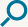Select a song:

# Let it be

[C]    [G]    [Am]    [F]
[C]    [G]    [F]    [C/E]    [Dm7]    [C]
When I [C]find myself in [G]times of trouble,
[Am]Mother Mary [F]comes to me,
[C]Speaking words of [G]wisdom,
let it [F]be. [C/E]    [Dm7]    [C]

And [C]in my hour of [G]darkness,
She is [Am]standing right in [F]front of me,
[C]Speaking words of [G]wisdom,
let it [F]be. [C/E]    [Dm7]    [C]

Let it [Am]be, let it [G]be,
let it [F]be, let it [C]be.
Whisper words of [G]wisdom,
let it [F]be. [C/E]    [Dm7]    [C]

And [C]when the broken [G]hearted people
[Am]Living in the [F]world agree,
let it [F]be. [C/E]    [Dm7]    [C]

For [C]though they may be [G]parted,
There is [Am]still a chance that [F]they will see,
let it [F]be. [C/E]    [Dm7]    [C]

Let it [Am]be, let it [G]be,
let it [F]be, let it [C]be.
let it [F]be. [C/E]    [Dm7]    [C]

Let it [Am]be, let it [G]be,
let it [F]be, let it [C]be.
Whisper words of [G]wisdom,
let it [F]be. [C/E]    [Dm7]    [C]

[F]    [C/E]    [Dm7]    [C]    [Bb]    [F/A]    [G]    [F]    [C]
[F]    [C/E]    [Dm7]    [C]    [Bb]    [F/A]    [G]    [F]    [C]

[C]    [G]    [Am]    [F]
[C]    [G]    [F]    [C/E]    [Dm7]    [C]
[C]    [G]    [Am]    [F]
[C]    [G]    [F]    [C/E]    [Dm7]    [C]

Let it [Am]be, let it [G]be,
let it [F]be, let it [C]be.
Whisper words of [G]wisdom,
let it [F]be.  [C]    [Dm7]    [C]

And [C]when the night is [G]cloudy,
There is [Am]still a light that [F]shines on me,
[C]Shine until to[G]morrow,
let it [F]be. [C/E]    [Dm7]    [C]

I [C]wake up to the [G]sound of music,
[Am]Mother Mary [F]comes to me,
[C]speaking words of [G]wisdom,
let it [F]be. [C/E]    [Dm7]    [C]

Let it [Am]be, let it [G]be,
let it [F]be, let it [C]be.
let it [F]be. [C/E]    [Dm7]    [C]

Let it [Am]be, let it [G]be,
let it [F]be, let it [C]be.
let it [F]be. [C/E]    [Dm7]    [C]

Let it [Am]be, let it [G]be,
let it [F]be, let it [C]be.
Whisper words of [G]wisdom,
let it [F]be. [C/E]    [Dm7]    [C]

[F]    [C/E]    [Dm7]    [C]    [Bb]    [F/A]    [G]    [F]    [C]

## Chords

•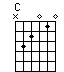•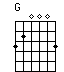•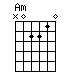••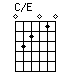••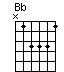•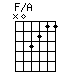•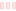Pick an instrument

Transpose the song